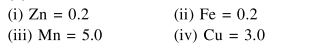Deepak Scored 45->99%ile with Bounce Back Crack Course. You can do it too!

# A water sample has ppm level concentration of the following metals:

Question:

A water sample has ppm level concentration of the following metals: $\mathrm{Fe}=0.2 ; \mathrm{Mn}=5.0 ; \mathrm{Cu}=3.0$; $\mathrm{Zn}=5.0$. The metal that makes the water sample unsuitable drinking is :

1. $\mathrm{Zn}$

2. $\mathrm{Fe}$

3. $\mathrm{Mn}$

4. $\mathrm{Cu}$

Correct Option: , 3

Solution: